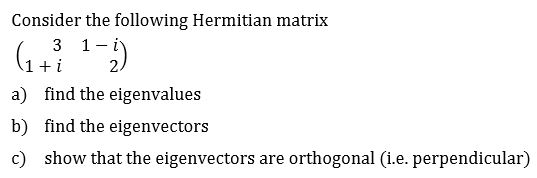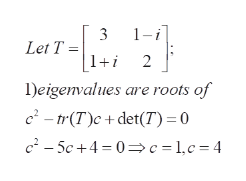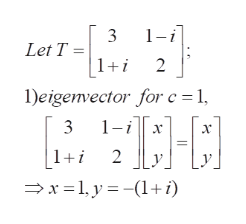# Consider the following Hermitian matrix1 i3ifind the eigenvaluesa)find the eigenvectorsb)show that the eigenvectors arec)orthogonal (i.e. perpendicular)

Question
1 viewshelp_outlineImage TranscriptioncloseConsider the following Hermitian matrix 1 i 3 i find the eigenvalues a) find the eigenvectors b) show that the eigenvectors are c) orthogonal (i.e. perpendicular) fullscreen
check_circle

Step 1

To find the eigenvalues, eigenvectors and establish the required orthogonallity

Step 2

1) eigenvalues are found by solving the characxteristic equation of Thelp_outlineImage Transcriptionclose1 -i 3 Let T = |1+i 2 Deigenvalues are roots of c2-tr(T)c+det(T) = 0 c2-5c+4 0c= 1,c =4 fullscreen
Step 3

2) eigenvector for c =1 is the column ...help_outlineImage Transcriptionclose1-i Let T |1+i 2 1)eigenvector for c 1 1-i 3 х х 2 x1y(1 i) fullscreen

### Want to see the full answer?

See Solution

#### Want to see this answer and more?

Solutions are written by subject experts who are available 24/7. Questions are typically answered within 1 hour.*

See Solution
*Response times may vary by subject and question.
Tagged in

### Math